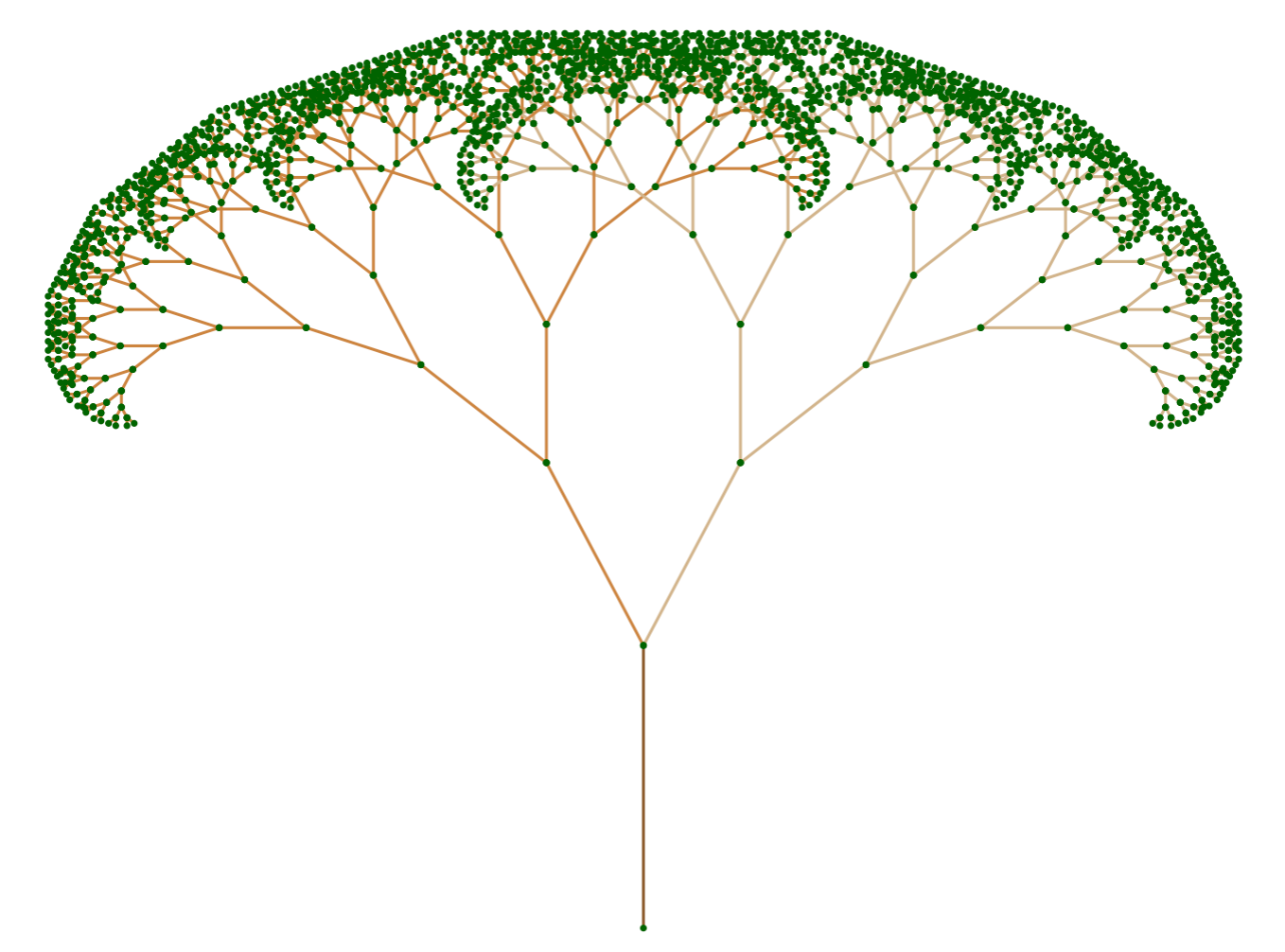Programmer/Data Scientist/Instructor・Mostly write Python & R・Big fan of OpenCV & p5js

Home

# OOP Fractal Trees in R with R6, ggplot2, & gganimate (part 1)

Published Oct 07, 2018

### Share

In this post, we’re going to explore OOP in R as implemented by the `R6` package. This post is part 1 of 2, and the end goal is to create an animated fractal tree with `R6` & `gganimate`. Today, we will be creating a static plot of a fractal tree and a series of `R6Class` objects to help get us there.

The below code and plot show where we’re going to get by the end of this post.

``````# Create & plot R6 tree object
tree = fractal_tree\$new()
tree\$plot()
``````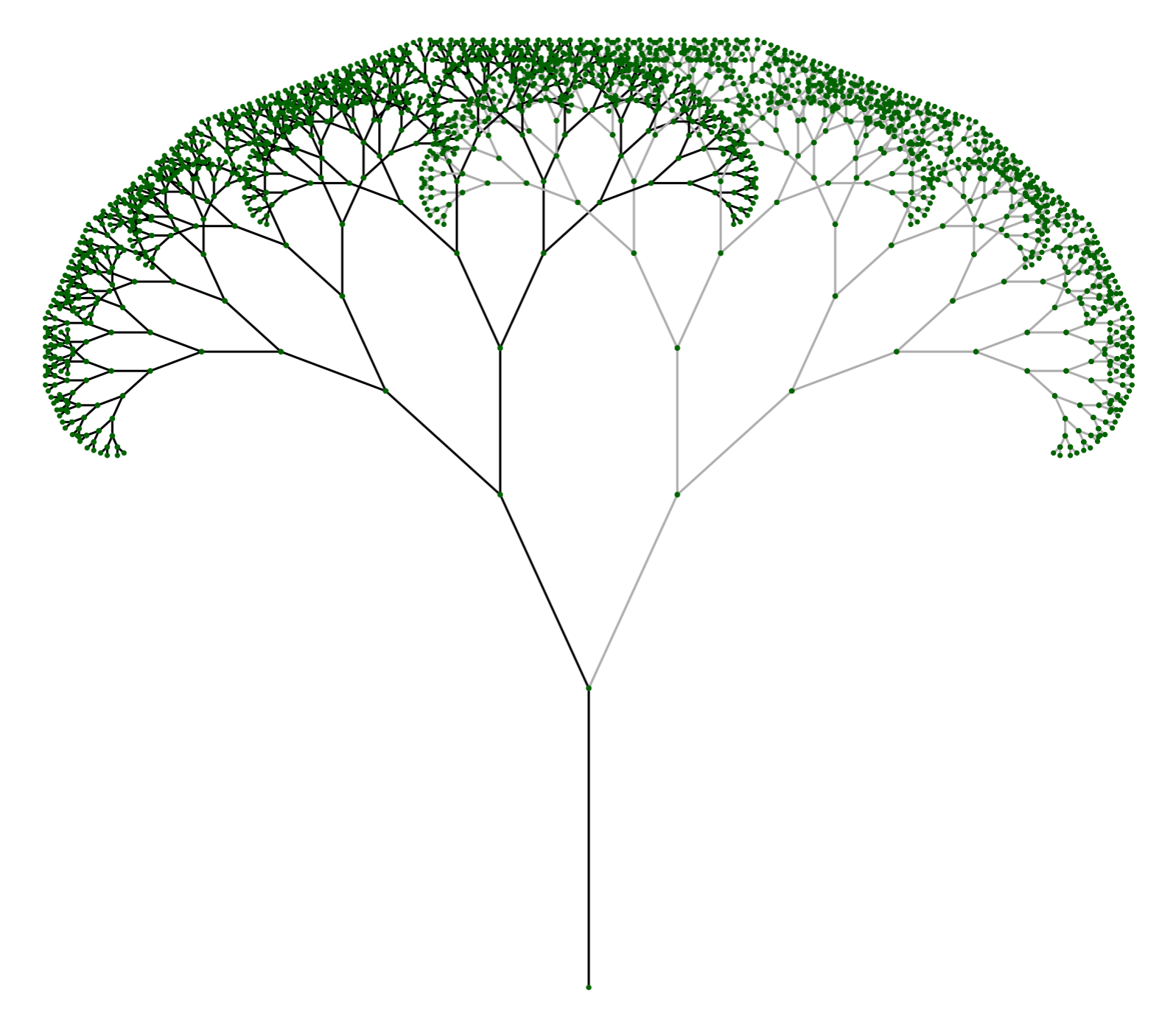Note: This post is meant to explore `R6` functionality; it’s not claiming to be the best way to create our fractal trees. Some design choices were solely to leverage varied features. Additionally, this post is more example-based than explanation based. For more in-depth explanations, I recomend going to this page from `R6` or check out this chapter from Advanced R

# Design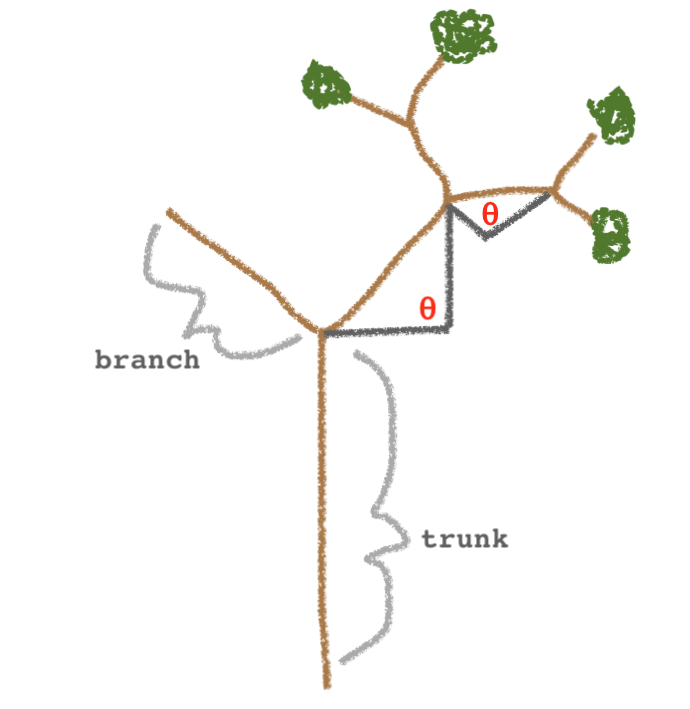The sketch above shows the basic design of the fractal tree we’ll be creating as an `R6` object; let’s unpack it. We’ll have a vertical line as the trunk, and a series of branch lines that recursively sprout two at a time. Lastly, each child branch will have the same angle relative to its parent branch.

Let’s translate the sketch into the object structure that we’ll be using.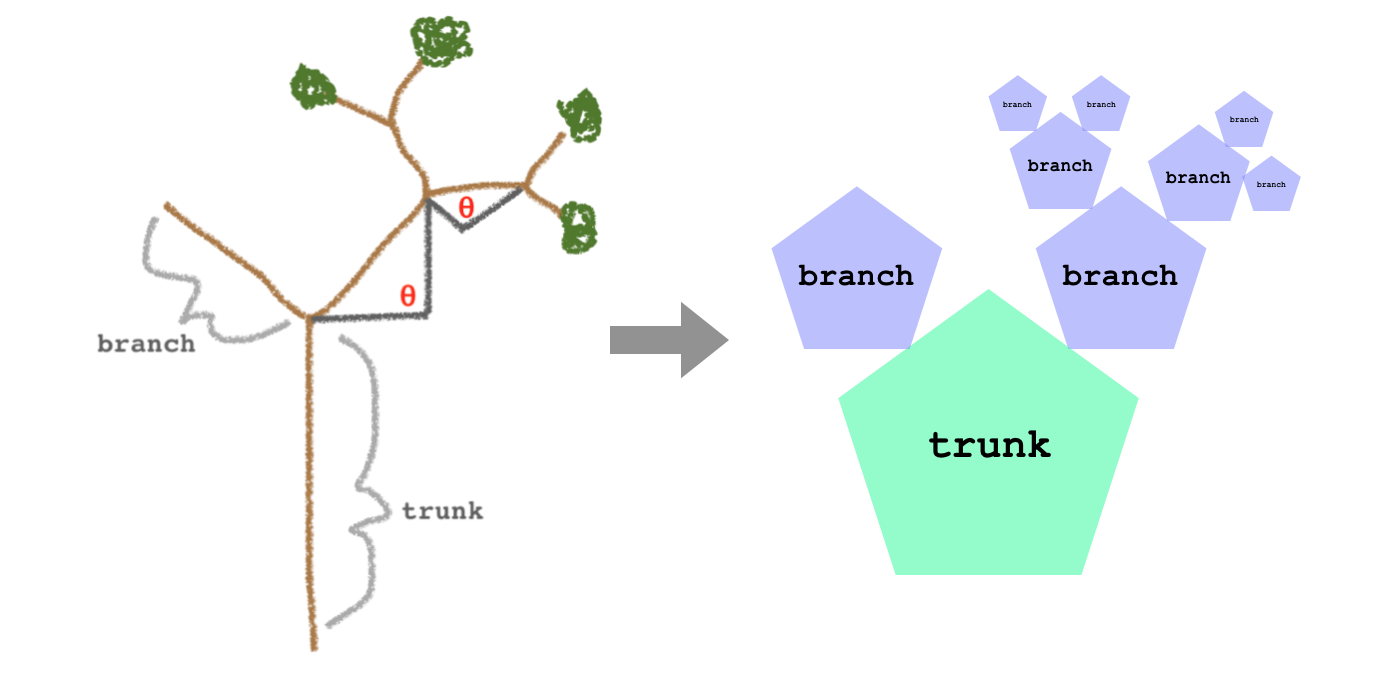The way it’s drawn up, we see that we’ll be using two separate classes for the trunk and branches. The trunk and branches have a lot in common, so we’ll be using the OOP concept of inheritance as implemented by `R6`. Below shows how we’ll implement the trunk and branch classes using a base class and inheritance to stay DRY.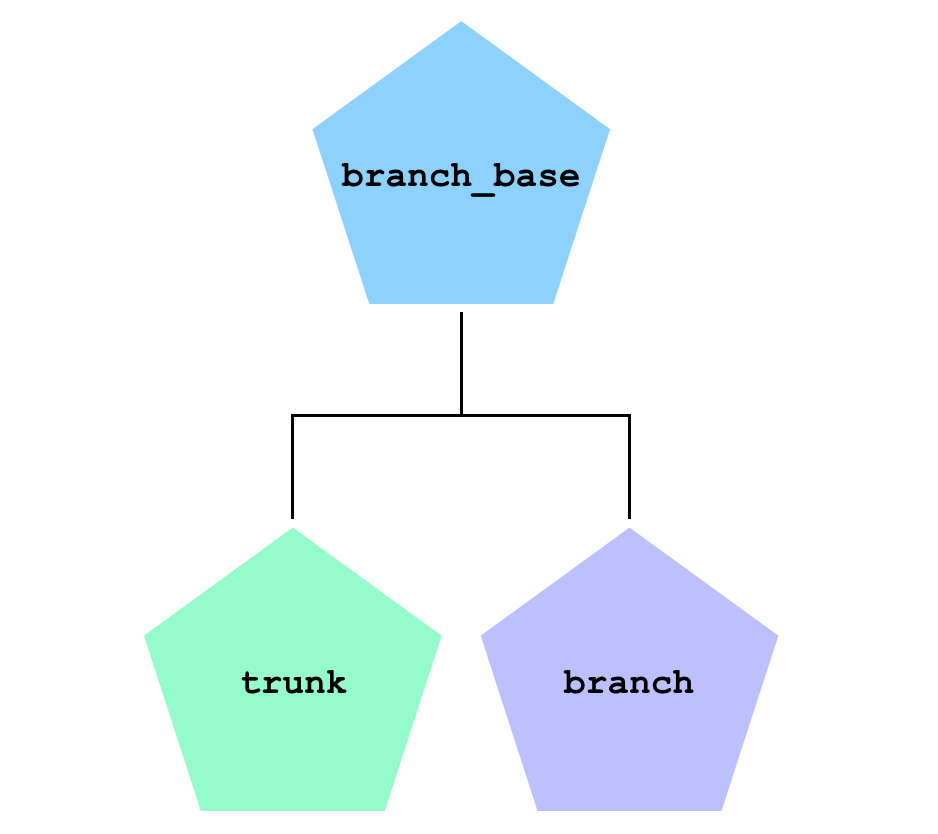# Implementation

We’ll be starting in reverse order of how things were laid out in the design section. The code will be broken out into different sections for easier digestion. If you want to see all the code in one place you can view it here.

## branch_base

The `branch_base` class will be the shared parent of our `trunk` & `branch` classes. So we want to pack it full of bits that they’ll share. From an implementation standpoint, what they share is how they’re going to be plotted by `ggplot2`. Each attribute that is initialized in `public` is an attribute that will be used to our plot method. Additionally, we use the `active` feature that will build a `data.frame` on the fly to represent our branches. Functions that are placed in `active` can be accessed as if they’re static attributes.

``````branch_base = R6Class('branch_base',
public = list(
start_x = NA_integer_,
start_y = NA_integer_,
end_x = NA_integer_,
end_y = NA_integer_,
type = NA_character_,
id = NA_character_,
color = NA_character_
),  # public

active = list(
df = function() {
x = c(self\$start_x, self\$end_x)
y = c(self\$start_y, self\$end_y)

data.frame(x = x, y = y,
type = self\$type,
id = self\$id,
branch_color = self\$color)
}
)  # active
)  # branch_base
``````

## trunk

The `trunk` class `inherit`s from `branch_base`, so we don’t have to think about initializing our attributes or redefining `active`. To define the class, we add an `initialize` method that will be used to actually create instances of `trunk`.

``````trunk = R6Class('trunk',
inherit = branch_base,
public = list(
initialize = function(len = 10, branch_color = '#000000') {
self\$start_x = 0
self\$start_y = 0
self\$end_x = 0
self\$end_y = len
self\$type = 'trunk'
self\$branch_color = branch_color

self\$id = uuid::UUIDgenerate()
}
)  # public
)  # trunk
``````

## branch

The definition of `branch` is similar to `trunk`, but a little more involved. When we need to create a `branch`, the info that we’ll be given is where it should start, what direction it should go in, and how long it should be. With these bits of information, we can use some trig to get the `branch`’s endpoint. The rest of the `initialize` method is very similar to `trunk`.

``````branch = R6Class('branch',
inherit = branch_base,
public = list(
initialize = function(x, y, len = 5, theta = 45,
type = NA_character_,
branch_color='#000000') {
dy = sin(theta) * len
dx = cos(theta) * len

self\$start_x = x
self\$start_y = y
self\$end_x = x + dx
self\$end_y = y + dy

self\$type = type
self\$id = uuid::UUIDgenerate()
self\$branch_color = branch_color
}
)  # public
)  # branch
``````

## fractal_tree

With `trunk` and `branch` defined we have the building blocks for our `fractal_tree` class. This class definition is going to be broken up into sections due to its length/complexity; the full definition can be seen here.

### public

The `public` section of `fractal_tree` consists of the functionality we need to create and plot our tree.

The `initialize` method creates all the branches of our tree including the trunk. The `private\$grow_branches` method is a recursive `private` method of our class that we’ll define soon.

The remaining `public` method is `plot`, which, unsurprisingly, will plot our tree. The contents of this method should look fairly familiar to those who are familiar with `ggplot2`. Thanks to our set up we are able to plot our tree with relatively little effort.

``````public = list(
delta_angle = NA_real_,
len_decay = NA_real_,
min_len = NA_real_,
branch_left_color = NA_character_,
branch_right_color = NA_character_,
branches = data.frame(),

initialize = function(trunk_len = 10,
delta_angle = pi / 8,
len_decay = 0.7,
min_len = 0.25,
trunk_color = '#000000',
branch_left_color = '#000000',
self\$delta_angle = delta_angle
self\$len_decay = len_decay
self\$min_len = min_len
self\$branch_left_color = branch_left_color
self\$branch_right_color = branch_right_color

self\$branches = trunk\$new(trunk_len, trunk_color)\$df

private\$grow_branches(0, trunk_len,
len = trunk_len * len_decay,
angle_in = pi / 2)
},

plot = function() {
ggplot(tree\$branches, aes(x, y, group = id, color=branch_color)) +
geom_line() +
geom_point(color = 'darkgreen', size=0.5) +
scale_color_identity() +
guides(color = FALSE, linetype = FALSE) +
theme_void()
}
), # public
``````

### private

Our `private` section consists of a single method, `grow_branches`. This method will recursively build out our tree forever if given a starting point and an angle. To avoid infinite recursion we’ve built in the `min_len` attribute that will serve as a stopping point.

The body of the function consists of:

• Creating 2 new branches that branch off to the left and right
• Adding these branches to the `branches` attribute (the way this `data.frame` is dynamically grown could be re-written to be more efficient)
• Repeating the process for the left branch (this recursively creates the entire left side of the tree)
• Repeating the process for the right branch (this recursively creates the entire right side of the tree)

And that’s it! We now finally have all the pieces in place to create and plot a `fractal_tree` with `R6` and `ggplot2`.

``````private = list(
grow_branches = function(start_x, start_y,
len = 1,
angle_in = pi / 2,
parent_type = NA,
parent_color = NA) {
if (len >= self\$min_len) {
l_type = if (!is.na(parent_type)) parent_type else 'left'
r_type = if (!is.na(parent_type)) parent_type else 'right'
l_color = if (!is.na(parent_color)) parent_color else self\$branch_left_color
r_color = if (!is.na(parent_color)) parent_color else self\$branch_right_color

branch_left  = branch\$new(start_x, start_y, len, angle_in + self\$delta_angle, l_type, l_color)
branch_right = branch\$new(start_x, start_y, len, angle_in - self\$delta_angle, r_type, r_color)

self\$branches = rbind(self\$branches,
branch_left\$df,
branch_right\$df)

private\$grow_branches(branch_left\$end_x,
branch_left\$end_y,
angle_in = angle_in + self\$delta_angle,
len = len * self\$len_decay,
parent_type = branch_left\$type,
parent_color = branch_left\$branch_color)

private\$grow_branches(branch_right\$end_x,
branch_right\$end_y,
angle_in = angle_in - self\$delta_angle,
len = len * self\$len_decay,
parent_type =  branch_right\$type,
parent_color = branch_right\$branch_color)
}
}  # grow_branches
)  # private
``````

# Final Product

This last section will be a few examples of using the functionality of our `fractal_tree` class.

``````# Create & plot R6 tree object
tree = fractal_tree\$new()
tree\$plot()
````````````# Create & plot R6 tree object with new angle
tree = fractal_tree\$new(delta_angle = pi / 3)
tree\$plot()
``````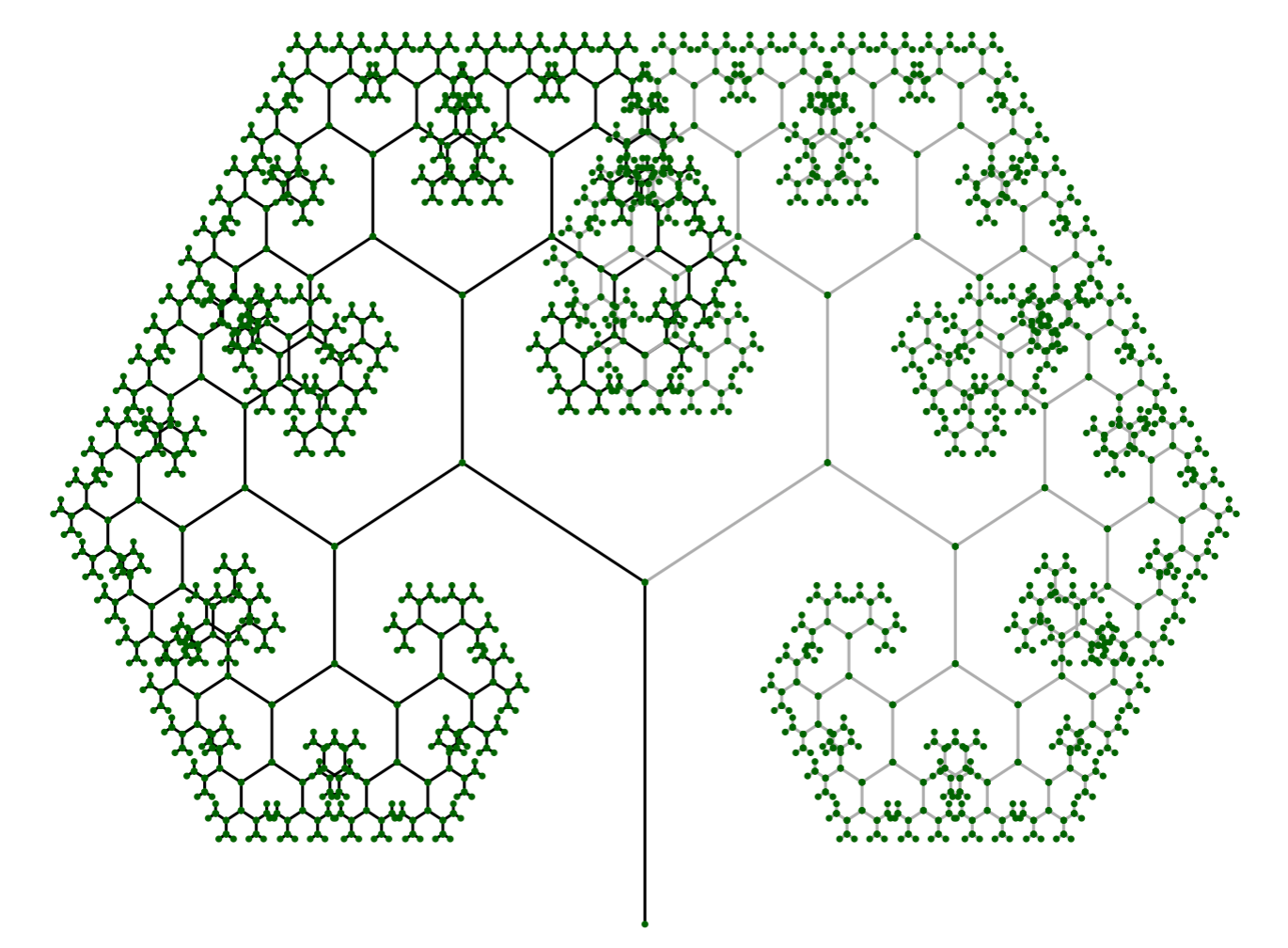``````# Create & plot R6 tree object with new branch length decay
tree = fractal_tree\$new(delta_angle = pi / 2,
len_decay = 0.6)
tree\$plot()
``````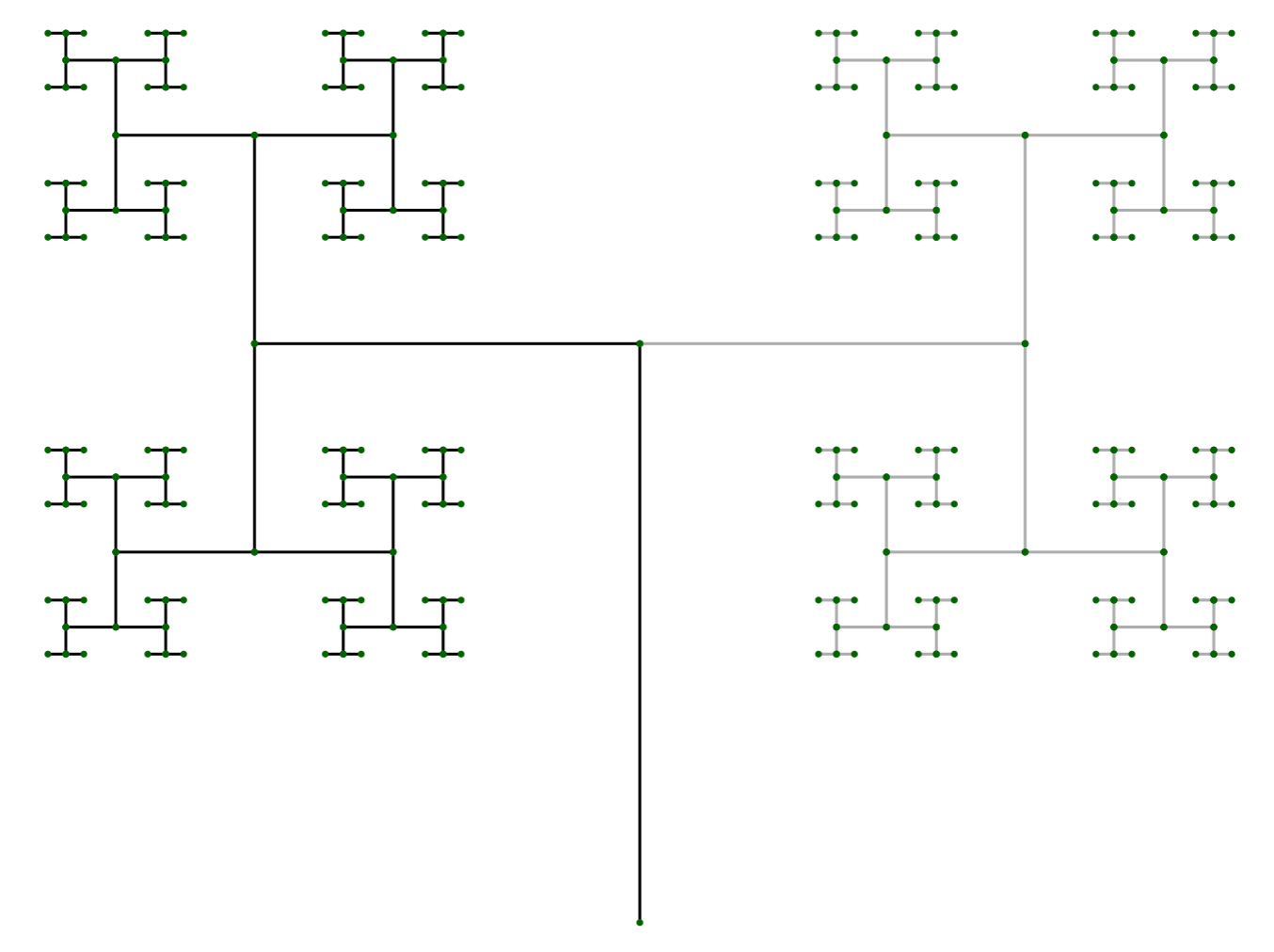``````# Create & plot R6 tree object with new color
tree = fractal_tree\$new(trunk_color = 'tan4',
branch_left_color = 'tan3',
branch_right_color = 'tan')
tree\$plot()
``````